## C. Turbulent Mixing Parameterization

The effects of subgrid scale turbulence are implemented using the parameterization of Klemp and Wilhelmson (1978) . In this parameterization, turbulent mixing coefficients are diagnosed from the turbulent kinetic energy that is predicted by a prognostic equation.

### Turbulent kinetic energy and turbulent mixing coefficient for momentum

The turbulent mixing coefficient for momentum Km is diagnosed from the turbulent kinetic energy E.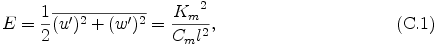where Cm = 0.2, l = (Δ x Δ z)1/2. Δ x, Δ z are grid intervals in the x and z directions, respectively. By using eq. (C.1), the prognostic equation of the turbulent kinetic energy E can be expressed in terms of the turbulent mixing coefficient for momentum.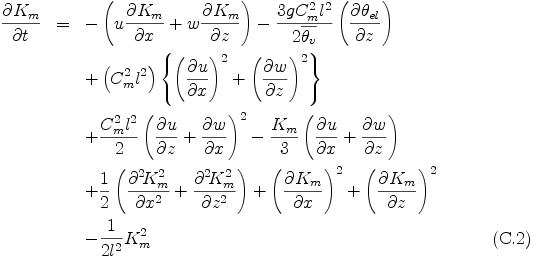where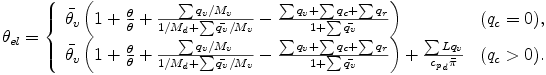For the sake of numerical stability, the value of Km is assumed to be bounded, and its maximum value is set to 800 m2s-1 .

### Expressions of turbulent mixing terms

The turbulent momentum mixing terms in eq. (A.4) and eq. (A.5) are now given by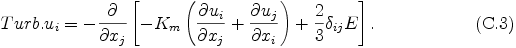The turbulent mixing terms in eq. (A.7) and eq. (A.8) are given by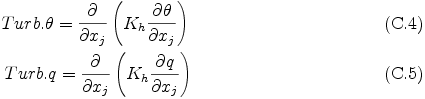where Kh is the turbulent mixing coefficient for scalar variables that is estimated from Km by the following relationship,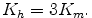### Dissipative heating in thermodynamic equation

Heating due to dissipation in eq. (A.7) is given by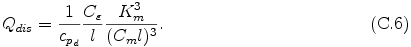where Cε = Cm = 0.2.